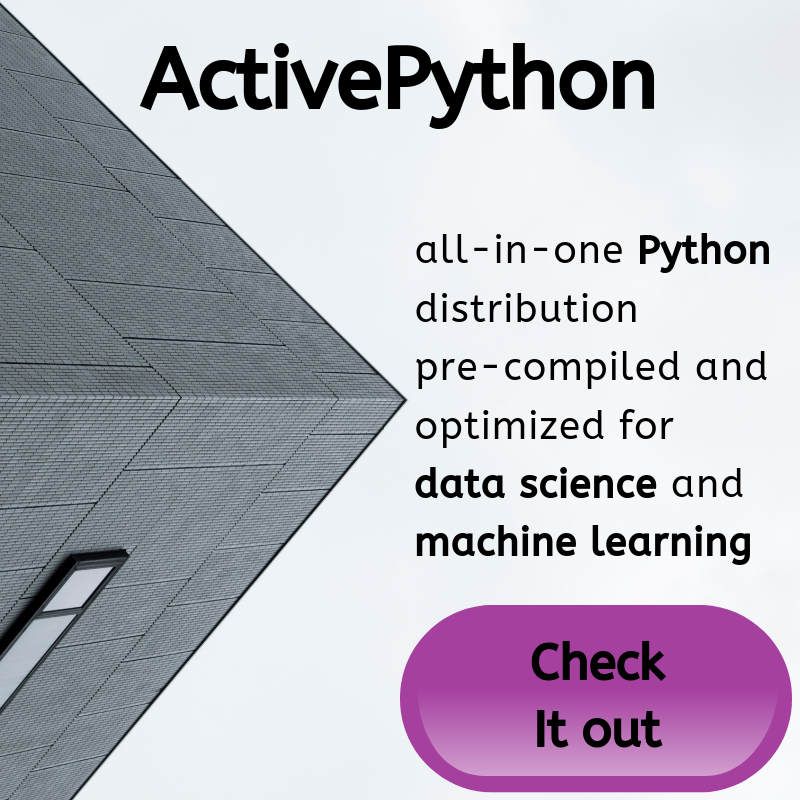## Buffering Data - Creating a Chatbot with Deep Learning, Python, and TensorFlow Part 3

Hello and welcome to part 3 of the chatbot with Python and TensorFlow tutorial series. In the last tutorial, we talked about the structure of our data and created a database to house our data. Now we're ready to begin working through the data!

Code up to this point:

```import sqlite3
import json
from datetime import datetime

timeframe = '2015-05'
sql_transaction = []

connection = sqlite3.connect('{}.db'.format(timeframe))
c = connection.cursor()

def create_table():
c.execute("CREATE TABLE IF NOT EXISTS parent_reply(parent_id TEXT PRIMARY KEY, comment_id TEXT UNIQUE, parent TEXT, comment TEXT, subreddit TEXT, unix INT, score INT)")

if __name__ == '__main__':
create_table()```

Now, let's begin to buffer through the data. We'll also start a couple of counters for tracking progress over time:

```if __name__ == '__main__':
create_table()
row_counter = 0
paired_rows = 0

with open('J:/chatdata/reddit_data/{}/RC_{}'.format(timeframe.split('-'),timeframe), buffering=1000) as f:
for row in f:```

The `row_counter` will just output from time to time to let us know how far we are in the file that we're iterating through, and then `paired_rows` will tell us how many rows of data we have that are paired (meaning we have comment and reply pairs, which are training data). Note that, of course, your path to the actual data file will be different than mine.

Next, because the file is too large for us to be dealing with in memory, we're going to use the `buffering` parameter, so we read the file in small chunks that we can easily work with, which is fine since all we care about is 1 line at a time.

Now, we need to read this row, which is of the json format:

```if __name__ == '__main__':
create_table()
row_counter = 0
paired_rows = 0

with open('J:/chatdata/reddit_data/{}/RC_{}'.format(timeframe.split('-'),timeframe), buffering=1000) as f:
for row in f:
row_counter += 1
parent_id = row['parent_id']
body = format_data(row['body'])
created_utc = row['created_utc']
score = row['score']
comment_id = row['name']
subreddit = row['subreddit']```

Note the `format_data` function call, let's create that:

```def format_data(data):
data = data.replace('\n',' newlinechar ').replace('\r',' newlinechar ').replace('"',"'")
return data```

We'll throw this in to normalize the comments and to convert the newline character to a word.

We can read the data into a python object by using `json.loads()`, which just takes a string formatted like a json object. As mentioned before, all comments will initially not have a parent, either because it's a top level comment (and the parent is the reddit post itself), or because the parent isn't in our document. As we go through the document, however, we will find comments that do have parents that we've got in our database. When this happens, we want to instead add this comment to the existing parent. Once we've gone through a file, or a list of files, we'll take the database and output our pairs as training data, train our model, and finally have a friend we can talk to! So, before we input our data to the database, we should see if we can find the parent first!

`            parent_data = find_parent(parent_id)`

Now, we need to create the `find_parent` function:

```def find_parent(pid):
try:
sql = "SELECT comment FROM parent_reply WHERE comment_id = '{}' LIMIT 1".format(pid)
c.execute(sql)
result = c.fetchone()
if result != None:
return result
else: return False
except Exception as e:
#print(str(e))
return False```

There's probably a more efficient way to do this, but this will do. So, if we have a `comment_id` in our database that matches another comment's `parent_id`, then we should match this new comment with the parent that we have already. In the next tutorial, we're going to begin building the logic required to determine whether or not to insert data, and how.

The next tutorial:• Creating a Chatbot with Deep Learning, Python, and TensorFlow Part 1

• Chat Data Structure - Creating a Chatbot with Deep Learning, Python, and TensorFlow Part 2

• Buffering Data - Creating a Chatbot with Deep Learning, Python, and TensorFlow Part 3
• Insert Logic - Creating a Chatbot with Deep Learning, Python, and TensorFlow Part 4

• Building Database - Creating a Chatbot with Deep Learning, Python, and TensorFlow Part 5

• Training Dataset - Creating a Chatbot with Deep Learning, Python, and TensorFlow Part 6

• Training a Model - Creating a Chatbot with Deep Learning, Python, and TensorFlow Part 7

• Exploring concepts and parameters of our NMT Model - Creating a Chatbot with Deep Learning, Python, and TensorFlow Part 8

• Interacting with our Chatbot - Creating a Chatbot with Deep Learning, Python, and TensorFlow Part 9